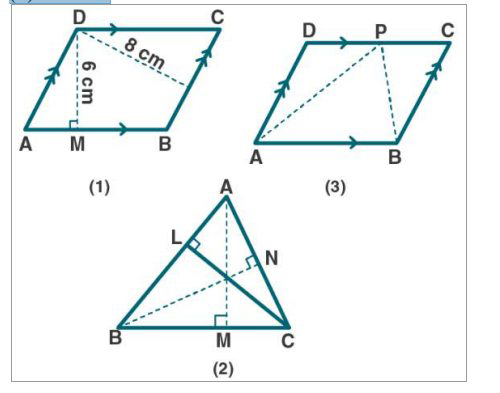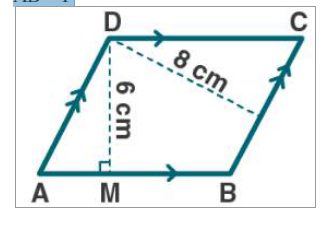# ML Aggarwal Solutions Class 9 Mathematics Solutions for Theorems on Area Exercise 14 in Chapter 14 - Theorems on Area

(a) In figure (1) given below, the perimeter of the parallelogram is 42 cm.

Calculate the lengths of the sides of the parallelogram.

(b) In the figure (2) given below, the perimeter of ∆ ABC is 37 cm. If the lengths of

the altitudes AM, BN and CL are 5x, 6x, and 4x respectively, Calculate the lengths

of the sides of ∆ABC.

(c) In the fig. (3) Given below, ABCD is a parallelogram. P is a point on DC such

that area of ∆DAP = 25 cm² and area of ∆BCP = 15 cm². Find

(i) area of || gm ABCD

(ii) DP: PC.(a) Given:

The perimeter of parallelogram ABCD = 42 cm

To find:

Lengths of the sides of the parallelogram ABCD.

From fig (1)

We know that,

AB = PThen, perimeter of ||gm ABCD = 2 (AB + BC)

42 = 2(P + BC)

42/2 = P + BC

21 = P + BC

BC = 21 – P

So, ar (||gm ABCD) = AB × DM

= P × 6

= 6P ………. (1)

Again, ar (||gm ABCD) = BC × DN

= (21 - P) × 8

= 8(21 - P) ………. (2)

From (1) and (2), we get

6P = 8(21 - P)

6P = 168 – 8P

6P + 8P = 168

14P = 168

P = 168/14

= 12

Hence, sides of ||gm are

AB = 12cm and BC = (21 - 12)cm = 9cm

(b) Given:

The perimeter of ∆ ABC is 37 cm. The lengths of the altitudes AM, BN and CL are 5x,

6x, and 4x respectively.

To find:

Lengths of the sides of ∆ABC. i.e., BC, CA, and AB.

Let us consider BC = P and CA = Q

From fig (2),

Then, perimeter of ∆ABC = AB + BC + CA

37 = AB + P + Q

AB = 37 – P – Q

Area (∆ABC) = ½ × base × height

= ½ × BC × AM = ½ × CA × BN = ½ × AB × CL

= ½ × P × 5x = ½ × Q × 6x = ½ (37 – P – Q) × 4x

= 5P/2 = 3Q = 2(37 – P – Q)

Let us consider first two parts:

5P/2 = 3Q

5P = 6Q

5P – 6Q = 0 …… (1)

25P – 30Q (multiplying by 5)….. (2)

Let us consider the second and third parts:

3Q = 2(37 – P – Q)

3Q = 74 – 2P – 2Q

3Q + 2Q + 2P = 74

2P + 5Q = 74 ……. (3)

12P + 30Q = 444 (multiplying by 6)……. (4)

By adding (2) and (4), we get

37P = 444

P = 444/37

= 12

Now, substitute the value of P in equation (1), we get

5P – 6Q = 0

5(12) – 6Q = 0

60 = 6Q

Q = 60/6

= 10

Hence, BC = P = 12cm

CA = Q = 10cm

And AB = 37 – P – Q = 37 – 12 – 10 = 15cm

(c) Given:

ABCD is a parallelogram. P is a point on DC such that area of ∆DAP = 25 cm² and the area

of ∆BCP = 15 cm².

To Find:

(i) area of || gm ABCD

(ii) DP: PC

Now let us find,

From fig (3)

(i) we know that,

ar (∆APB) = ½ ar (||gm ABCD)

Then,

½ ar (||gm ABCD) = ar (∆DAP )

= 25 + 15

\begin{array}{l} =40 \mathrm{cm}^{2} \\ \text { So, ar }(\| \mathrm{gm} \mathrm{ABCD})=2 \times 40=80 \mathrm{cm}^{2} \end{array}

(ii) we know that,

∆ADP and ∆BCP are on the same base CD and between the same parallel lines CD and AB.

ar (∆DAP)/ar(∆BCP) = DP/PC

25/15 = DP/PC

5/3 = DP/PC

So, DP: PC = 5: 3

Related Questions
Exercises

Lido

Courses

Teachers

Book a Demo with us

Syllabus

Maths
CBSE
Maths
ICSE
Science
CBSE

Science
ICSE
English
CBSE
English
ICSE
Coding

Terms & Policies

Selina Question Bank

Maths
Physics
Biology

Allied Question Bank

Chemistry
Connect with us on social media!